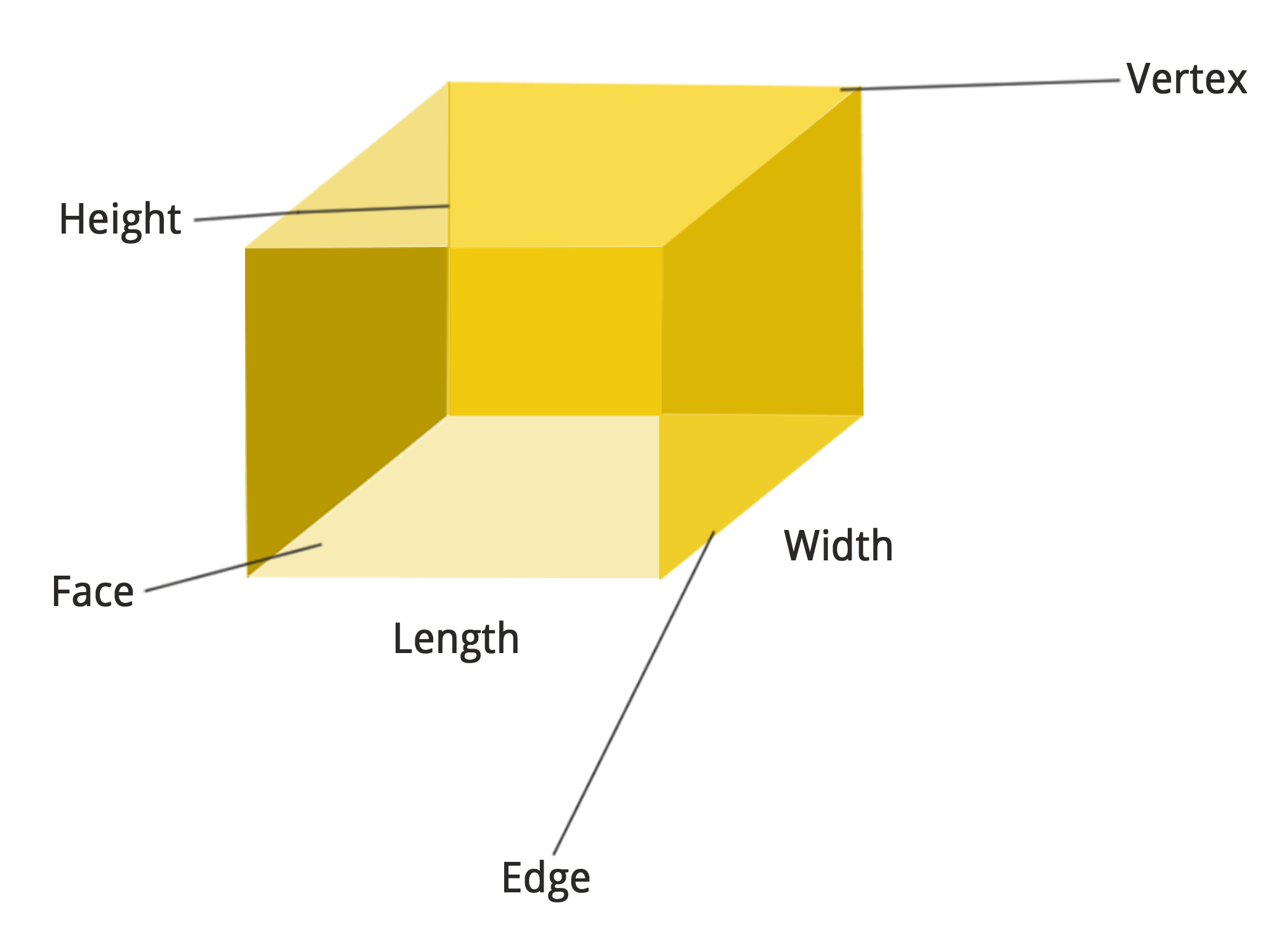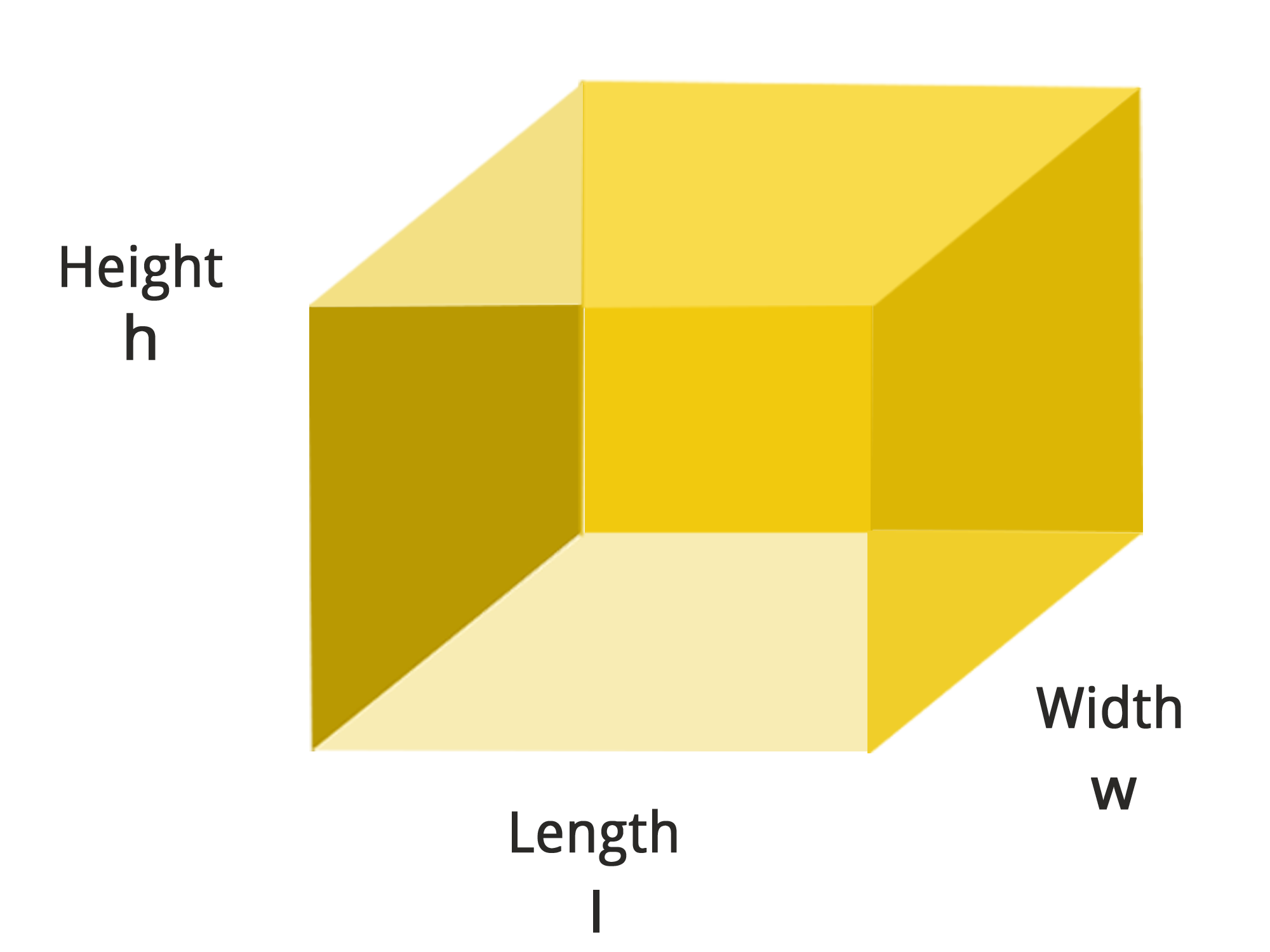• Call Now

1800-102-2727•

# Cuboid and Cube

A cuboid can be seen as a three-dimensional shape with length, height, and width. Cuboids have six sides. These sides are known as countenances. Each cuboid face is shaped in a rectangle, and the majority of corners of the cuboid make 90° edges. They can also be termed vertices. In simple terms, a cuboid is nothing but a closed rectangular box.Following are some real-time examples of a cuboid; a table drawer, a book, a cell phone etc. Some of the cuboid related formulae are given below;

The perimeter of a cuboid:
Perimeter = 4 * (L + H + W)

To identify complete edge to edge separation, one should use this perimeter formula.
The volume of cuboid:
Volume = L * H * W cubic units

The volume of a cuboid is the total amount of space occupied by the cuboid in a 3-dimensional space. Volume is usually measured in cubic units.
The Lateral Surface Area of a Cuboid:
Lateral Surface Area = 2 [(L * H) + (W * H)] square units

The lateral area of the surface is nothing but the area of the appearances along with the height.
The Total Surface Area of a Cuboid:
Total Surface Area = 2 [(W * H) + (L * H) + (W * H)] square units

The total surface area is equal to the total of the areas of all six rectangular sides.

## Cube

Cube is a geometrical shape in mathematics, a three-dimensional figure containing 6 square faces, eight vertices and 12 edges. The cube can be termed a regular hexahedron. All six sides of the cube are equal. Usually, the shape of the cube is considered similar to a square box. One can also say that a cube is considered a block in which it has all the length, height, and breadth equal. A cube is also termed as a right rhombohedron, a square parallelepiped or an equilateral cuboid. The cube can be seen as one of the platonic solids and considered the convex polyhedron where its faces are square. One can also say that a cube has octahedral or cubical symmetry. Cube is a special case in a square prism.Given below are the cube related formulae,
The surface area of a cube is determined as the region occupied by it in a specific plane. A cube is a 3-dimensional object. The occupied area will be in a 3D plane. Since the cube has six equal faces, in order to calculate the surface area of the cube, the following formula is used;

Lateral Surface Area = 4 * Area of one face

LSA = 4 a2 square units

The formula for total surface area is given below:
Total Surface Area = LSA + Area of top and bottom faces
TSA = 4 a2 + a2 + a2
TSA = 6 a2 square units

In lateral surface area calculation, the top and bottom faces are eliminated, whereas, in the total calculation, all the faces are taken into account.

The volume of the cube is nothing but the amount of space contained in it. The formula for this is,

The volume of cube = a3 cubic units

The real-life examples of a cube are 3 * 3 Rubik’s cube, 6 sided dice etc.Talk to our expert
Resend OTP Timer =
By submitting up, I agree to receive all the Whatsapp communication on my registered number and Aakash terms and conditions and privacy policy